# Advanced VLOOKUP in Excel: multiple, double, nested

These examples will teach you how to Vlookup multiple criteria, return a specific instance or all matches, do dynamic Vlookup in multiple sheets, and more.

It is the second part of the series that will help you harness the power of Excel VLOOKUP. The examples imply that you know how this function works. If not, it stands to reason to start with the basic uses of VLOOKUP in Excel.

Before moving further, let me briefly remind you the syntax:

VLOOKUP(lookup_value, table_array, col_index_num, [range_lookup])

Now that everyone is on the same page, let's take a closer look at the advanced VLOOKUP formula examples:

## How to Vlookup multiple criteria

The Excel VLOOKUP function is really helpful when it comes to searching across a database for a certain value. However, it lacks an important feature - its syntax allows for just one lookup value. But what if you want to look up with several conditions? There are a few different solutions for you to choose from.

### Formula 1. VLOOKUP with two criteria

Suppose you have a list of orders and want to find the quantity based on 2 criteria, Customer name and Product. A complicating factor is that each customer ordered multiple products, as shown in the table below: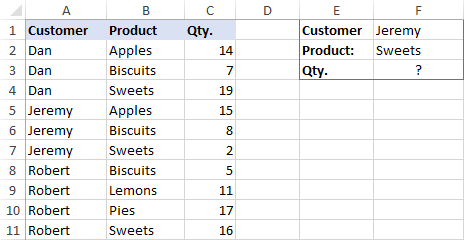A usual VLOOKUP formula won't work in this situation because it returns the first found match based on a single lookup value that you specify.

To overcome this, you can add a helper column and concatenate the values from two lookup columns (Customer and Product) there. It is important that the helper column should be the leftmost column in the table array because it's where Excel VLOOKUP always searches for the lookup value.

So, add a column to the left of your table and copy the below formula across that column. This will populate the helper column with the values from columns B and C (the space character is concatenated in between for better readability):

`=B2&" "&C2`

And then, use a standard VLOOKUP formula and place both criteria in the lookup_value argument, separated with a space:

`=VLOOKUP("Jeremy Sweets", A2:D11, 4, FALSE)`

Or, input the criteria in separate cells (G1 and G2 in our case) and concatenate those cells:

`=VLOOKUP(G1&" "&G2, A2:D11, 4, FALSE)`

As we want to return a value from column D, which is fourth in the table array, we use 4 for col_index_num. The range_lookup argument is set to FALSE to Vlookup an exact match. The screenshot below shows the result: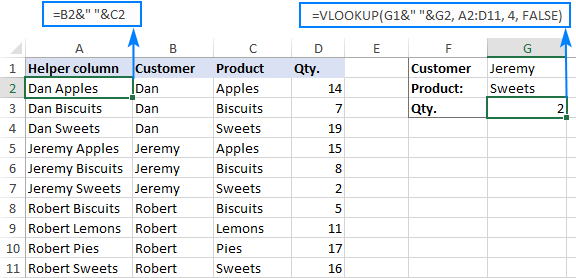In case your lookup table is in another sheet, include the sheet's name in your VLOOKUP formula. For example:

`=VLOOKUP(G1&" "&G2, Orders!A2:D11, 4, FALSE)`

Alternatively, create a named range for the lookup table (say, Orders) to make the formula easier-to-read:

`=VLOOKUP(G1&" "&G2, Orders, 4, FALSE)`

Note. For the formula to work correctly, the values in the helper column should be concatenated exactly the same way as in the lookup_value argument. For example, we used a space character to separate the criteria in both the helper column (B2&" "&C2) and VLOOKUP formula (G1&" "&G2).

### Formula 2. Excel VLOOKUP with multiple conditions

In theory, you can use the above approach to Vlookup more than two criteria. However, there are a couple of caveats. Firstly, a lookup value is limited to 255 characters, and secondly, the worksheet's design may not allow adding a helper column.

Luckily, Microsoft Excel often provides more than one way to do the same thing. To Vlookup multiple criteria, you can use either an INDEX MATCH combination or the XLOOKUP function recently introduced in Office 365.

For example, to look up based on 3 different values (Date, Customer name and Product), use one of the following formulas:

`=INDEX(D2:D11, MATCH(1, (G1=A2:A11) * (G2=B2:B11) * (G3=C2:C11), 0))`

`=XLOOKUP(1, (G1=A2:A11) * (G2=B2:B11) * (G3=C2:C11), D2:D11)`

Where:

• G1 is criteria 1 (date)
• G2 is criteria 2 (customer name)
• G3 is criteria 3 (product)
• A2:A11 is lookup range 1 (dates)
• B2:B11 is lookup range 2 (customer names)
• C2:C11 is lookup range 3 (products)
• D2:D11 is the return range (quantity)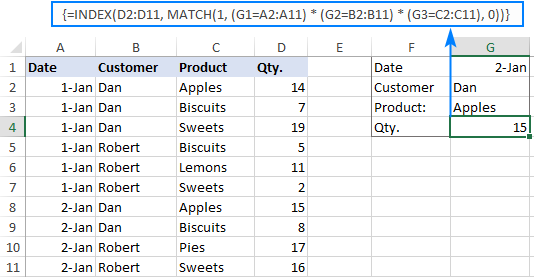Note. In all versions except Excel 365, INDEX MATCH should be entered as an CSE array formula by pressing Ctrl + Shift + Enter. In Excel 365 that supports dynamic arrays it also works as a regular formula.

For the detailed explanation of the formulas, please see:

## How to use VLOOKUP to get 2nd, 3rd or nth match

As you already know, Excel VLOOKUP can fetch only one matching value, more precisely, it returns the first found match. But what if there are several matches in your lookup array and you want to get the 2nd or 3rd instance? The task sounds quite intricate, but the solution does exist!

### Formula 1. Vlookup Nth instance

Suppose you have customer names in one column, the products they purchased in another, and you are looking to find the 2nd or 3rd product bought by a given customer.

The simplest way is to add a helper column to the left of the table like we did in the first example. But this time, we will populate it with customer names and occurrence numbers like "John Doe1", "John Doe2", etc.

To get the occurrence, use the COUNTIF function with a mixed range reference (the first reference is absolute and the second is relative like \$B\$2:B2). Since the relative reference changes based on a position of the cell where the formula is copied, in row 3 it will become \$B\$2:B3, in row 4 - \$B\$2:B4, and so on.

Concatenated with the customer name (B2), the formula takes this form:

`=B2&COUNTIF(\$B\$2:B2, B2)`

The above formula goes to A2, and then you copy it down to as many cells as needed.

After that, input the target name and occurrence number in separate cells (F1 and F2), and use the below formula to Vlookup a specific occurrence:

`=VLOOKUP(F1&F2, A2:C11, 3, FALSE)`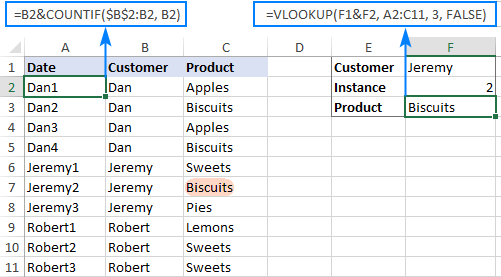### Formula 2. Vlookup 2nd occurrence

If you are looking for the 2nd instance of the lookup value, then you can do without the helper column. Instead, create the table array dynamically by using the INDIRECT function together with MATCH:

`=VLOOKUP(E1, INDIRECT("A"&(MATCH(E1, A2:A11, 0)+2)&":B11"), 2, FALSE)`

Where:

• E1 is the lookup value
• A2:A11 is the lookup range
• B11 is the last (bottom-right) cell of the lookup table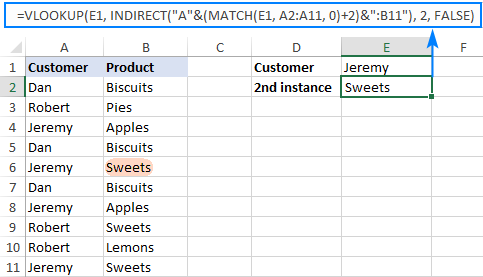Please note that the above formula is written for a specific case where data cells in the lookup table begin in row 2. If your table is somewhere in the middle of the sheet, use this universal formula, where A1 is the top-left cell of the lookup table containing a column header:

`=VLOOKUP(E1, INDIRECT("A"&(MATCH(E1, A2:A11, 0)+1+ROW(A1))&":B11"), 2, FALSE)`

#### How this formula works

Here is the key part of the formula that creates a dynamic vlookup range:

`INDIRECT("A"&(MATCH(E1, A2:A11, 0)+2)&":B11")`

The MATCH function configured for exact match (0 in the last argument) compares the target name (E1) against the list of names (A2:A11) and returns the position of the first found match, which is 3 in our case. This number is going to be used as the starting row coordinate for the vlookup range, so we add 2 to it (+1 to exclude the first instance and +1 to exclude row 1 with the column headers). Alternatively, you can use 1+ROW(A1) to calculate the necessary adjustment automatically based on the position of the header row (A1 in our case).

As the result, we get the following text string, which INDIRECT converts to a range reference:

`INDIRECT("A"&5&":B11") -> A5:B11`

This range goes to the table_array argument of VLOOKUP forcing it to start searching in row 5, leaving out the first instance of the lookup value:

`VLOOKUP(E1, A5:B11, 2, FALSE)`

## How to Vlookup and return multiple values in Excel

The Excel VLOOKUP function is designed to return just one match. Is there a way to Vlookup multiple instances? Yes, there is, though not an easy one. This requires a combined use of several functions such as INDEX, SMALL and ROW is an array formula.

For example, the below can find all occurrences of the lookup value F2 in the lookup range B2:B16 and return multiple matches from column C:

`{=IFERROR(INDEX(\$C\$2:\$C\$11, SMALL(IF(\$F\$1=\$B\$2:\$B\$11, ROW(\$C\$2:\$C\$11)-1,""), ROW()-1)),"")}`

There are 2 ways to enter the formula in your worksheet:

1. Type the formula in the first cell, press Ctrl + Shift + Enter, and then drag it down to a few more cells.
2. Select several adjacent cells in a single column (F1:F11 in the screenshot below), type the formula and press Ctrl + Shift + Enter to complete it.

Either way, the number of cells in which you enter the formula should be equal to or larger than the maximum number of possible matches.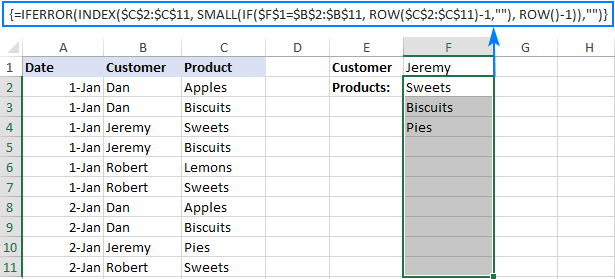For the detailed explanation of the formula logic and more examples, please see How to VLOOKUP multiple values in Excel.

## How to Vlookup in rows and columns (two-way lookup)

Two-way lookup (aka matrix lookup or 2-dimentional lookup) is a fancy word for looking up a value at the intersection of a certain row and column. There are a few different ways to do two-dimensional lookup in Excel, but since the focus of this tutorial is on the VLOOKUP function, we will naturally use it.

For this example, we'll take the below table with monthly sales and work out a VLOOKUP formula to retrieve the sales figure for a specific item in a given month.

With item names in A2:A9, month names in B1:F1, the target item in I1 and the target month in I2, the formula goes as follows:

`=VLOOKUP(I1, A2:F9, MATCH(I2, A1:F1, 0), FALSE)`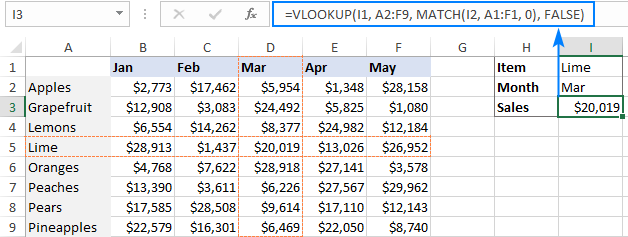### How this formula works

The core of the formula is the standard VLOOKUP function that searches for an exact match to the lookup value in I1. But since we do not know in which exactly column the sales for a specific month are, we cannot supply the column number directly to the col_index_num argument. To find that column, we use the following MATCH function:

`MATCH(I2, A1:F1, 0)`

Translated into English, the formula says: look up the I2 value in A1:F1 and return its relative position in the array. By supplying 0 to the 3rd argument, you instruct MATCH to find the value exactly equal to the lookup value (it's like using FALSE for the range_lookup argument of VLOOKUP).

Since Mar is in the 4th column in the lookup array, the MATCH function returns 4, which goes directly to the col_index_num argument of VLOOKUP:

`VLOOKUP(I1, A2:F9, 4, FALSE)`

Please pay attention that although the month names start in column B, we use A1:I1 for the lookup array. This is done in order for the number returned by MATCH to correspond to the column's position in table_array of VLOOKUP.

To learn more ways to perform matrix lookup in Excel, please see INDEX MATCH MATCH and other formulas for 2-dimensional lookup.

## How to do multiple Vlookup in Excel (nested Vlookup)

Sometimes it may happen that your main table and lookup table do not have a single column in common, which prevents you from doing a Vlookup between two tables. However, there exists another table, which does not contain the information you are looking for but has one common column with the main table and another common column with the lookup table.

In below image illustrates the situation: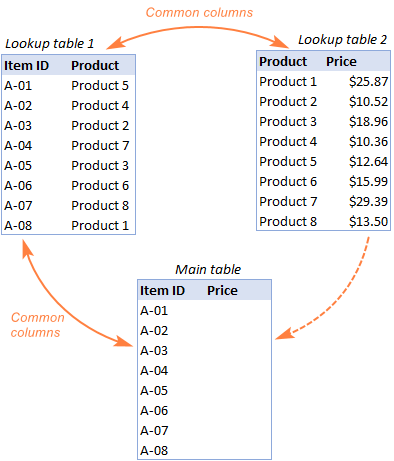The goal is to copy prices to the main table based on Item IDs. The problem is that the table containing prices does not have the Item IDs, meaning we will have to do two Vlookups in one formula.

For the sake of convenience, let's create a couple of named ranges first:

• Lookup table 1 is named Products (D3:E10)
• Lookup table 2 is named Prices (G3:H10)

The tables can be in the same or different worksheets.

And now, we will perform the so-called double Vlookup, aka nested Vlookup.

First, make a VLOOKUP formula to find the product name in the Lookup table 1 (named Products) based on the item id (A3):

`=VLOOKUP(A3, Products, 2, FALSE)`

Next, put the above formula in the lookup_value argument of another VLOOKUP function to pull prices from Lookup table 2 (named Prices) based on the product name returned by the nested VLOOKUP:

`=VLOOKUP(VLOOKUP(A3, Products, 2, FALSE), Prices, 2, FALSE)`

The screenshot below shows our nested Vlookup formula in action: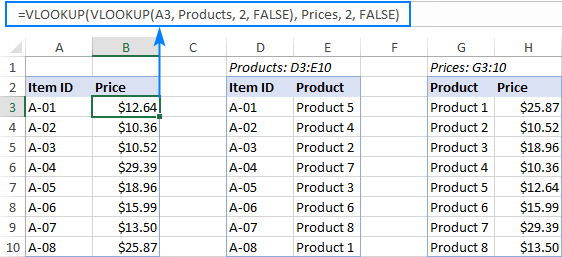## How to Vlookup multiple sheets dynamically

Sometimes, you may have data in the same format split over several worksheets. And your aim is to pull data from a specific sheet depending on the key value in a given cell.

This may be easier to understand from an example. Let's say, you have a few regional sales reports in the same format, and you are looking to get the sales figures for a specific product in certain regions: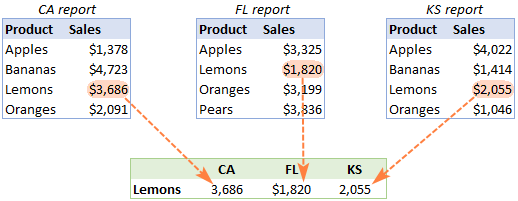Like in the previous example, we start with defining a few names:

• Range A2:B5 in CA sheet is named CA_Sales.
• Range A2:B5 in FL sheet is named FL_Sales.
• Range A2:B5 in KS sheet is named KS_Sales.

As you can see, all the named ranges have a common part (Sales) and unique parts (CA, FL, KS). Please be sure to name your ranges in a similar manner as it's essential for the formula we are going to build.

### Formula 1. INDIRECT VLOOKUP to dynamically pull data from different sheets

If your task is to retrieve data from multiple sheets, a VLOOKUP INDIRECT formula is the best solution – compact and easy-to-understand.

For this example, we organize the summary table in this way:

• Input the products of interest in A2 and A3. Those are our lookup values.
• Enter the unique parts of the named ranges in B1, C1 and D1.

And now, we concatenate the cell containing the unique part (B1) with the common part ("_Sales"), and feed the resulting string to INDIRECT:

`INDIRECT(B\$1&"_Sales")`

The INDIRECT function transforms the string into a name that Excel can understand, and you put it in the table_array argument of VLOOKUP:

`=VLOOKUP(\$A2, INDIRECT(B\$1&"_Sales"), 2, FALSE)`

The above formula goes to B2, and then you copy it down and to the right.

Please pay attention that, in the lookup value (\$A2), we've locked the column coordinate with absolute cell reference so that the column remains fixed when the formula is copied to the right. In the B\$1 reference, we locked the row because we want the column coordinate to change and supply an appropriate name part to INDIRECT depending on the column into which the formula is copied: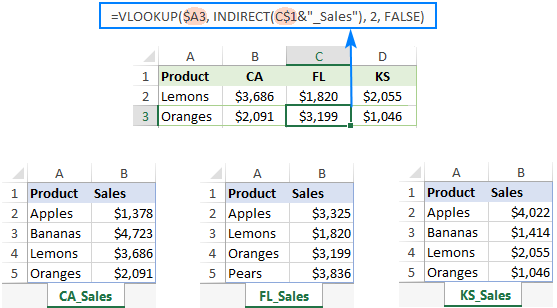If your main table is organized differently, the lookup values in a row and unique parts of the range names in a column, then you should lock the row coordinate in the lookup value (B\$1) and the column coordinate in the name parts (\$A2):

`=VLOOKUP(B\$1, INDIRECT(\$A2&"_Sales"), 2, FALSE)`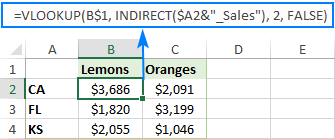### Formula 2. VLOOKUP and nested IFs to look up multiple sheets

In situation when you have just two or three lookup sheets, you can use a fairly simple VLOOKUP formula with nested IF functions to select the correct sheet based on the key value in a particular cell:

`=VLOOKUP(\$A2, IF(B\$1="CA", CA_Sales, IF(B\$1="FL", FL_Sales, IF(B\$1="KS", KS_Sales,""))), 2, FALSE)`

Where \$A2 is the lookup value (item name) and B\$1 is the key value (state):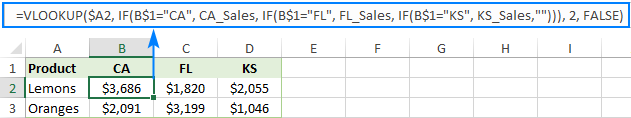In this case, you do not necessarily need to define names and can use external references to refer to another sheet or workbook.

For more formula examples, please see How to VLOOKUP across multiple sheets in Excel.

That's how to use VLOOKUP in Excel. I thank you for reading and hope to see you on our blog next week!

Advanced VLOOKUP formula examples (.xlsx file)

## You may also be interested in

1. Hello,

Im trying to find a vlookup that will return when a value is found in the column (when there is no blank). For example:

item
item
item value1

VLOOKUP should return "value1", not 0 or blank. How can I accomplish? Thank you.

• Hello!
VLOOKUP function will not be able to solve your problem. Use INDEX + MATCH in formula:

=INDEX(B2:B20,MATCH(TRUE,(A2:A20="item")*(B2:B20 > 0) > 0,0))

• Unfortunately this only returns me #N/A. Is there another way?

• Found the issue. It needs to be shift + ctrl + entered :)

2. Dear Mam

=IF(C8<711,5.12,) this is being charged as working days
Do you an idea that 6.14 will be charged on weekend days instead of 5.12 in the same formula.

Thanks

• Hi,
The information you provided is not enough to understand your case and give you any advice.
To check a weekday or a weekend, use the WEEKDAY function.

3. Is there any way to allocate product based on available production to meet model wise item target with minimum and maximum quantity per day? thanks in advance.

4. I have 2 excel sheets, both containing EAN of products. 1st sheet has 9000 EANs while 2nd sheet has 7000 EANs. 1st sheet have same 7000 EANs same as in 2nd sheet but remaining 2000 are not common in both sheet. Please let me know how can I find which 2000 EANs are not in 2nd sheet using Vlookup command.

5. SKU _XS _S _M _L _XL _2X _3XL Total
Os103 10 10 10 10 10 10 10 70

I have a data in this way but i want to convert this excel data this format

SKU Qty
Os103_XS 10
Os103_S 10
Os103_M 10
Os103_L 10
Os103_XL 10
Os103_2XL 10
Os103_3XL 10
I have try to some formula in Excel Like Vlookup and &&
Any one can help ??

6. I am trying to complete a project for work to calculate BMI and I'm not sure how I should go about it. This is what I've accomplished thus far:
CELL
C2 = height (inches, I used mine which is 77)
C3 = weight (lbs, I used mine which is 210)
C4 = BMI (=703*\$C\$3/\$C\$2^2) this returns a 24.90 rounded up slightly with .00 formatting
C5 = BMI Status

C5 is where I am experiencing difficulties. I have a separate table for a BMI chart in the same sheet which encompasses H2:J6. H2:J2 Row are my column headers for the table (BMI Low Range, BMI High Range, BMI Status). Below is my chart data:
Cell
H3 = 0 (BMI Low Range)
I3 = <18.5 (BMI High Range)
J3 = Underweight

H4 = 18.5 (BMI Low Range)
I4 = <25 (BMI High Range)
J4 = Normal

H5 = 25 (BMI Low Range)
I5 = <30 (BMI High Range)
J5 = Overweight

H6 = 30 (BMI Low Range)
I6 = 200 (BMI High Range)
J6 = Obese

What I'm trying to accomplish is taking the calculated result from C4 and comparing it to the BMI chart. The corresponding BMI status in the chart (ie J3:J6) will display in C5.
=IF(COUNTIF(\$C\$4,H3&"<"&I3),J3)& IF(COUNTIF(\$C\$4,H4&"<"&I4),J4)& IF(COUNTIF(\$C\$4,H5&"="&H6),J6)

All it returns is FALSEFALSEFALSEFALSE... with the 24.90 calculated result I have in C5 now it should return at a minimum FALSETRUEFALSEFALSE or FALSENORMALFALSEFALSE right? I'm thinking that vlookup may be what I need but am unsure.

Any help would be greatly appreciated and thank you for your time.

V/r,
James

• Hello!
If I understand your task correctly, the following formula should work for you:

=VLOOKUP(C4,H3:J6,3,1)

I hope this will help, otherwise please do not hesitate to contact me anytime.

7. Hello, please make a correction:
Formula 2.
=INDEX(D2:D11, MATCH(1, (G1=A2:A11) * (G2=B2:B11) * (G3=C2:C11), 0))
Needs to be entered as array formula with Ctrl+Shift+Enter

{=INDEX(D2:D11, MATCH(1, (G1=A2:A11) * (G2=B2:B11) * (G3=C2:C11), 0))}

Thx! Nice article!

• Hi Alexandr,

Thank you for pointing that out! In Excel 365 that I am using it works as a regular formula due to support for dynamic arrays, and I completely forgot about older versions, sorry for that. I've added a note about Ctrl + Shift + Enter. Thank you!

8. Use your mouse to enter an IF function that displays a value of “Yes” if the Stock Qty field (cell F5) is less than or equal to the Reorder Qty field (cell H5) and “No” if it is not.

9. Hi,

Do you know how can I use the lookup value of 2000 parameters?

Thanks,
Mariecris

• Hi Mariecris,

You can use an INDEX MATCH formula instead of VLOOKUP. It does not have a limit to the size of the lookup value.

10. i have same reference number- under muliple data's, i should take vlookup in other excel. how to take?pls help

11. How to find same names same code persons are duplicate
Ex:-
1. Naresh 000
2. ABC 212
3. naresh 000
4. ABC 212

12. Hello,
I want to run a daily manning report from an excel roster but don't know how to go about it. I want it to be set up as classifications and Manning Numbers and then have total of each classifications and so it has to provide having personnel showing as on isolations from the current manning as well.
Can you help me?

13. i have C collumn Col-A,Col-B & Col-C
Under Col-A 4 Data(A1=Name,A2=Ram,A3=Ram,A4=Ram)
Under Col-A 4 Data(B1=Salary,B2=Blank,B3=Blank,B4=4000)
Under Col-A 4 Data(C1=Attendance,C2=Blank,C3=P,C4=A)
Suggest me formula by Vlookup & Index where i can get the data from Col B(Salary) & Col C(Attendance).after Blank.Name is same in A col when run the formula based on Name then form the array provide me 4000 from B col and P from C col. its meance it check data if found blank then move to second and at end provide me after blnk i case of same name.
alwasy provide me data after scape blank cell and swap next row.

Name Salary Attendace
Ram
Ram 4000
Heera A
Ram 3000 P
Heera 2000 A
Heera 1000 A
Ram 500 A

Name Salary
Ram 4000 Result always this

Name Attendace
Ram P Result always this

Name Salary
Heera 2000 Result always this

Name Attendace
Heera A Result always this

• Still i dont recive any solutions

14. Thank you for the detailed instruction for different uses of VLOOKUPs.
I'd like to find out if I could use VLOOKUP to look for a value when a condition applies in the lookup table. For example, =VLOOKUP(Lookup_value_list, lookup_table!A1:D100, 2, false) Under column B, let's say it has certain number of Value As and certain number of Value Bs, I want to add a condition (like a filter to the lookup_table) to ask the vlookup funtion to only look for Bs in the lookup_table. How do I do this without applying for a filter before hand? Thank you in advance!I would appreciate your insights!

15. Is it possible to import every Nth Cell from another Sheet. I am trying to import every 6th cell from Column C. I’ve tried doing this but I keep ending up with the imported data appearing every 6 rows. I then tried to sort the range this compiled the data but also alphabetised it which I don’t want. Thanks!

16. Hello,

I wanted to know on how can I pull data for a certain information like "XYZ" from the data provided below.

Item Set Code Test
ABC, IJK, RST, XYZ 5 2001 Major
ABC 2 251 Major
IJK 6 4001 Remission
RST 6 9002 Depression
XYZ 9 12003 Remission
IJK, XYZ 10 8009 Remission
ABC, RST 11 4007 Depression

Thanks

17. In the single cell we have multiple values like this CAA
CBG
ERT
HGJ
when i am trying vlookup 1st value (CAA) only coming , remaining values not come.

• Hello Thavakumar!
For me to be able to help you, please describe your problem in more detail. What values are there in the cells you are applying your formula to?
Are your 4 values typed in the same cell or in 4 different ones?
What formula are you using to look for values?
Please let me know. I think I can suggest a solution but some additional information is needed.

18. Hi i want use vlookup formula but i have issue to find out result with two lookup value result will be same
as a expamle :
THIS IS DATA
A B C
ORENGE FROUIT
APPLE FROUIT
POTATO VEG
TOMATO VEG

a b
1ST LOOKUP VALUE 2ND LOOKUP VALUE FIND OUT RESULT
ORENGE APPLE ????????

19. Good day, I am busy with big recons. I want to match info lines (A-X) with info lines (Y-AV) but my key codes is in G and AD). How do I do 'n v-lookup dat say "match code in G with Code in AD, if it matches in the same line 227 it must say yes and if it doesn't match in the same line it must say false. I hope I make sense.

20. thank you so much.

21. Hi
I need to vlookup (one column have part numbers) and
(another have part numbers in between - as separating 3 integers).
Eg. 12345678
123-456-78
but both are same.
Kindly assist me how to make it
Thanx

22. thank you so much.
you are my hero.

23. i want get multipal value in sheet like, Amir Have got Amount 5 time and 5 payment are in different Row vloook will got 1st amount and sumif will count All amount but i want like this
1st Amount 151
2nd Amount 515
3rd 120

24. Hey guys can teach me. If I have entire of Emp Name with staff Id and Date. How am I going to run the VBA excel in a diffent worksheet.

Thank you
All angel

• You can simply use this code
Sheets("Sheetname").range("Cell name").value
further i can teach you on team viewer.
+923220000671 is my whatsapp numebr.

25. Hi ,
Kindly Help With vlookup function of Below Criteria.
column A b
row 1 Type value
Row 2 Apple,mango = vlookup( ?? ) result should be 5

Data
column A b
row 1 Type value
Row 2 Apple 2
Row 3 mango 3

26. Hi! I have a large database (over 12,000 rows and A to AZ columns) of a name dictionary. It is in constant flux, as some details change or new names come up. I need to prepare for publication a worksheet of all those names that have a certain info in one of the columns. I could do that by filtering, but I would need to stop updating the main database. Can I somehow create a linked worksheet with the names that CURRENTLY have that info but that gets automatically updated if changes (more names or change of info) occur in the main database? I could create a dynamic table and do a Vlookup, but I would get all the empty rows as well, which I don't need.

27. Hello

I need a vlookup formula which searches for the most correct result.

The situation is as follows. I have a table of hundreds of cities, there cities have specific numbers given. I want ecxell to give me the number of Vienna Oldtown even if I just type Vienn or oldtown.

28. work book1
date card number reg amount
01/01/2019 1076206 60719 80.25
02/01/2019 1076206 60719 13.00

work book2
date card number reg amount
01/01/2019 1076206 60719 80.25
02/01/2019 1076206 60719 13.00
HOW TO RETURN THE CORRECT VALUE TO " WORK BOOK 1", if we used vlookup its return the first lookup value both card number. but it is wrong.

pls help

29. Hi,
I'm sharing few data as given below..request you to please share the solution for this.
Raw Data Lookup_Value OutPut
NYM1035/323141085 323141085 NYM1035/323141085
1218454/UEI00007463 1218454 1218454/UEI00007463
1219385/UEI00007485
399152/919095
1200053831628 / 1200054080311
399381/919186
918447 / RAHUL.U713051981455
TXPC5141 / 323177477
YASWANTHUPUDI123406121991198/918491
sgumparthi3410061970989/918559
OLESH0000000659482052639/1GPZ070050
QFAM5567 / 323338673
323370031 / RIWR2689
323425520 / BVFG6740
YMUE0863 // 323284372
XKTM6146 // 323295089
5021777833/919207
NFBW0723 / 323276824
399514 // dsankar00615081968136
919240 / kashishkumar201902071989968
397760 / ME.PRITAMGHOSH10061992935

30. Multiple latest 6 dates from name A1 dates B2 on sheet 1 vertically (Name appears over 20 times with multiple names same quality of times & dates appears over 100 times with multiple dates same quality of times ) to return on another sheet 2 with all information horizontal in formula of latest date / second latest date / third latest date / & so on.
Name is the start A1 to match latest date B2 CRITICAL for rest of information B to AW
Have Changed work sheet 2 with name to start from A1
Oldest Dates B2 start from top of sheet with latest date at the bottom & keep adding to list to bottom
Have used ; =Vlookup(A4,Section!A:B,2,false) — found old date. NOT LATEST
{=Max(if(section!A4:A20000=A4,Section!B4:B20000))}
=Min. As above.
=Arrayformula(Vlookup (A4,section!A4:B20000,2,false))

If I have space between one date to the next date does this matter as I have separated all following dates?

Sheet size is 30 columns A - AW & rows 1 - 50,000 plus will grow in size with added information down the sheet.

I hope you can help with this formula to match dates & 6 latest in order & the information to the right of these dates.?
Regards
Tony

31. dear, I need help. For Example, IF we have many entries against value "A" than how I can pick the latest date value using the formula in excel. and if I have many entries of different values how I can pick the latest date value. (Sorry For My English)

32. hey; i need your help; i have a sheet containing 500 types of equipment each equipment has 2 or three prices and i need to get from it the min price , average and max prices and returning the supplier name for each value

33. Hi, I'm trying to create a v-lookup formula that will look at the corresponding ID in column 1 of sheet1 (which contains my formula), then lookup the ID on sheet2 and bring in the data from column 7 of sheet2 IF... column 6 = 2019. I do v-lookups between sheets all the time and have been trying to put it together with an IF formula but I haven't been able to get it to work. Can you help?

i refer above all step for my case, but still not found a solution. i have 2 kind of excel sheet. when i select one date in 1st sheet need to get 2 detail from another sheet according to selection data. i try to lot of time & through the every step. but couldn'd. please help me....

35. Hi,
i am having some product in my master sheet. and i am having month wise sheet like sheet 1= jan,sheet2= fab,...now i enter randomly product name in month wise sheet and his corresponding value. for using vlookup formula i collect corresponding product value from sheet1= jan by(=VLOOKUP(A9,'jan'!B7:F26,5,0). so now i want to collect value for same product in other remaning month and adding value form all month in master sheet. please help

I've looked for a vlookup formula to add figures with common letter code down columns. Example (Letters col. A, \$Values col. B):

AAA \$330
AAA \$600
AAA \$270
BBB \$300
CCC \$100
CCC \$120

On my separate spreadsheet, I would like to add all AAA values in one cell, \$1,200; all BBB \$300; CCC \$220, etc.

Thanks!

37. Hi,

How can i get different variable with same answer
For example

Name Roll nol
amit 1
vikas 2
ajeet 3
raj 4
manish 5
sanjeet 6
suraj 7
amit 8

38. My data is given below:

A B C D
Product Rohim korim jodu
TV 100 200 300
AC 301 302 303
FREEZER 3002 3005 3009

After enter the following data I need result (?)
A B C
Rohim TV ??? (result should be 100)
Korim AC ??? (result should be 302)

How can I do it with vlookup or other formula in column C . Pls help.

39. hi
i am learning excel and i am using a football table,what i`m stuck on is when i enter the weeks results i now want to be able to look for a teams name and be able to bring up its last 5 results to show its current form,i can do this with a pivot table but its time consuming doing every team i was wondering if it can be done to show the whole leagues current form

thanks
colin

40. Hi,
In the below formula what is the ! symbol for?
=LOOKUP(D26,'B:\HPServices\Current\[Material Price Book.xlsx]Material Pricing'!\$B2:\$B196,'B:\HPServices\Current\[Material Price Book.xlsx]Material Pricing'!\$H2:\$H196)

Brian

41. =IF(ISNA(MATCH(A2, Individual!\$A\$2:\$A\$108385, 0)), VLOOKUP(A2, Individual!\$A\$2:\$A\$108385, C2&"/"&C3, FALSE)," ")

Row ID Relationship Name
132361 Father Buchi Ramulu
132361 Mother Sujatha
132364 Father Mahesh
132387 Father B.Ramulu
132387 Mother Kondamma
132390 Father Anjaneyulu
132390 Mother Laxmamma

This Row ID should match with other sheet Row ID and return father & mother name "Father/Mother" in single cell.

• =CONCATENATE(B2&C2) 132361 Father Buchi Ramulu 132361 Father =VLOOKUP(CONCATENATE(F2&G2),A:D,4,0) Mother =VLOOKUP(CONCATENATE(F2&I2),A:D,4,0) =CONCATENATE(H2,"/",J2)
1. in other sheet, do text to column. Put Unique ID and Father and Mother in col.
2. First look up( concatenate father and id and mother and id) in Raw Data and look up through Lookup.
3. then concatenate mother and father.

42. HiHi,
I have query regarding find/search and vlookup.
I want find specific text from cell (string) and retrieve data from vlookup table by using this specific text.
e.g. “This is a boy” or “This is a girl” is in one cell.(there will more than 100 sentences) In Vlookup table Boy = Male and girl = Female.
I want display Male or Female in another cell of same sheet depends on cell string contains boy or girl.
Regards,

43. need a formula in vlookup for 2 scenario

44. Can Someone please advise me, how can i have value of one label appearing multiple times in Col-A and its return value in Col-B

For Ex

Col-A Col-B
ABC 1
ABC 2
ABC 3
XYZ 4
CBA 5
ABC 6
ABC 7
FFF 8

to other sheet like below

ABC 1
ABC 2
ABC 3
ABC 6
ABC 7

Thanks
Sudip

45. Vlookup to get 2nd occurrence using Indirect function is not working. It's giving the first occurrence only. It's working in the sample file attached. But it's not working in real time scenarios.

46. =VLOOKUP(B\$1,RT,2,)
Kindly Explain

47. how to concatenate a coloumn data from A1:A10 without using formula =CONCATENATE(A1&A2&A3&A4&A5&A6&A7&A8&A9&A10)

IS THERE ANY OTHER FORMULA FOR GOT CONCATENATE OF A1TOA10 CELLS VALUE AT ONCE

• you can use cocat function in excel2019

48. Hi Ablebits!
Thanks Very much! Your tutorials do me great job!
My vlookup dosent work out, it returns N/A i have tried all trouble shootslike advised.
Question; Can the version of excel be an issue, besides does the fomat of the cell be a matter.
waiting
Ronald

• Hi Ronald,

Vlookup works in all versions of Excel, but the format of the cell can be an issue, for example a number formatted as text. You can find a list of the most common reasons for #N/A and other errors in this tutorial:
Excel VLOOKUP not working

49. How do we check using vlookup which customer ordered Apple

Column A = Customer
Row 1A= John
Row 2A= Susan
Row 3A= Sherry

Column B = Product
Row 1B= Grapes,Apple
Row 2B= Apples,Lime
Row 3B= Pear,Pineapple

50. HI

I have an excel data like following, i want to retrieve style DEC and PQR becasue all their SKU's had sale. do not want to retrieve data for styles whose all SKU's did not have sale.

Style SKU Sale
ABC 111
ABC 222 Yes
ABC 333 Yes
ABC 444 Yes
ABC 555 Yes
DEF 1111 Yes
DEF 2222 Yes
DEF 3333 Yes
DEF 4444 Yes
DEF 5555 Yes
PQR 121 Yes
PQR 212 Yes
PQR 312 Yes
PQR 412 Yes
PQR 512 Yes
PQR 612 Yes
PQR 712 Yes
LMN 322 Yes
LMN 422 Yes
LMN 522 Yes
LMN 622
LMN 722